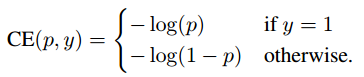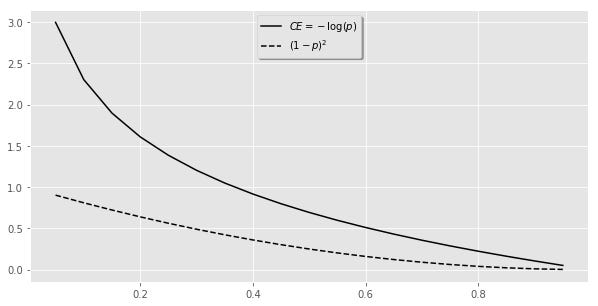Lazy Neural Networks

For difficult problems neural networks can sometimes lack robustness whereby they might fail to perform accurate predictions on under classified examples and edge cases. This can still be the case even if suitable architectures have been selected. I discuss how focusing attention away from model architecture and more towards intelligent data selection strategies and cost function design is often a more useful strategy.

Before I get into solutions I think it is important to discuss some overarching themes of deep learning.

Training Objectives

Remember that when we create a neural network, what we are effectively doing is designing an experiment. We have data, a model architecture and a training objective. The data you provide is the models universe and the loss function is basically how the neural network evaluates itself against this objective. This last point is critical. The loss function basically provides feedback to the architecture during training effectively stating – Is the current state of the parameters within the architecture making a prediction that accurately reflects our training target, with lower numbers being better.

Data classification is a common problem to solve using deep learning. Whether you try to classify cats versus dogs, cars on a road or purchasing behavior. A training objective for such problems is to simply minimize the amount of misclassified examples. The current paradigm is to use a loss function called Cross-Entropy with the training objective to minimize the total output. Mathematically this is represented as:

Or in the first case, can be visually represented as:

Although most of you familiar with deep learning are probably sick of people explaining Cross-Entropy, I would like to highlight a few things. Look at the shape of the curve above and try to imagine what goes on during training. The parameters are randomly initialized and then updated as it passes through the data. Since a lower loss is better, if these randomly initialized weights result in a predicted probability p that is close to zero then the loss output will be extremely high (the curve tends to infinity). If the parameters are perfect such that it predicts everything in the training set with 100% accuracy then the output is zero and cannot get any lower. If it is at any intermediate location, it will always try to aim towards this value of zero. This is because our training objective is minimize the total loss, and the sum of lots of small values is better than the sum of lots of large values.

The above methodology seems like it needs no modification. We have a continuous function that tells the neural network to keep on trying to improve until it is perfect. I will come back to why this approach can be problematic in a moment.

Neural Networks Get Lazy

Data is critical for deep learning models. But concluding that more data is better would be a gross oversimplification. A small number of high quality examples can be more than enough to have an effective model. However, understanding the whole training process is important to have a robust model.

Neural networks are not initialized with common sense. They start with no concept of what is being learned, gradually updating parameters by looking at the data, which are then evaluated based on what gives the best overall performance, the criterion being the total loss which we have defined for it. Class imbalance can result in a network simply predicting the dominant class when it is unsure. Uncertainty itself can come from low variation in your data.

If you view data as the models universe, a large number of easy, well classified examples can reinforce a models belief that no such variation of that class even exists. For example, an object detector trained to identify people might struggle to locate a person at a distance and facing at an angle if all it was trained on was passport photos.

Even if you have some variability in your dataset, a large number of easy examples reinforces a networks belief about its universe. Consider again the Cross-Entropy Loss curve. A neural network may not attempt to try learn from difficult examples as the risk for its total loss is too high. The unfortunate consequence of this could be a network that says “do nothing”, “stand still” or “unknown”. In another case, imagine you are trying to train a network to play Rock, Paper, Scissors. It may learn that the majority of people always play rock and then perpetually output paper when in reality you want it to learn more complicated relationships. This is why simply adding more data is not always ideal as this notion could be further reinforced.

A more useful approach is to ask the question where does my neural network suck and make those cases more represented in your dataset. This idea is known as bootstrapping or hard negative mining. Computer vision has historically dealt with the issue of lazy models using this method. In object detection problems the background and foreground classes can be dominant on the scale of 1000:1 and never learn to focus learning on the objects themselves. The key idea was to gradually grow, or bootstrap , the set of background examples by selecting those examples for which the detector triggers a false alarm. This strategy leads to an iterative training algorithm that alternates between updating the detection model given the current set of examples, and then using the updated model to find new false positives to add to the boot-strapped training set. The process typically commences with a training set consisting of all object examples and a small, random set of background examples .

The takeaway here is that more data can be useful, but don’t simply throw it at your network and expect better results.

Mathematical Intuition

I wouldn’t argue that to be a good machine learning practitioner that you need extensive math skills, certainly not compared to programming or general problem solving skills. I wouldn’t even argue that you can’t read papers unless you understand the often cryptic mathematical explanations behind what is being demonstrated. However, I would say that being able to visualize recurring mathematical concepts in deep learning does provide another tool for you to use in both understanding why a neural network might not end up being robust and whether there is room for improvement.

When you read papers it can be hard to intuitively understand what is going on. Understanding some basic tricks to visualize mathematical functions can make it easier.

Let’s forget about deep learning functions for a moment and just imagine a simple sinusoid. The simplest modification one can do is to multiply it by a constant, which in this case is less than one. The effect is the same shape but the values are scaled.

Next imagine multiplying our sinusoid by a function which changes, in this case a simple line (y = x). A sinusoid normally oscillates between –1and 1. By multiplying it by another function it is now forced to oscillate between the positive and negative values of this function, -x and x in this case.

Focal Loss

If we remember that we are doing an experiment with three components consisting of data, architecture and a loss function; why not change the loss function in addition to the other two? If we don’t want our neural network to be lazy, how can we change this function? More specifically, how can we reduce the contribution of large number of easy examples so parameters aren’t refined to hone in on these examples? How can we force it to discover more complex relationships only present in the harder under-represented examples? By multiplying two functions together as demonstrated with the sinusoidal function, it should be possible to reshape Cross-Entropy Loss in such a way that it correctly costs examples the way we want.

This is what the Focal Loss function achieves. It was first introduced in the paper Focal Loss for Dense Object Detection (2017) to solve the problem of class imbalance in computer vision as a simpler alternative to hard negative mining and multi-staged models. Basically, the goal was to identify objects in images with huge class imbalance between the background and foreground classes relative to the object classes one is trying to predict. Basically it was difficult to get networks to even attempt to classify the objects as a neural network deems the risk in terms of loss too high.

Focal loss simply multiplies Cross-Entropy Loss by a scaling factor which decays to zero as confidence in the correct class increases .

If we represent Cross-Entropy Loss and the scaling factor separately:Cross-Entropy Loss and the scaling factor for case when gamma is 2

And now together, while comparing it with the unscaled version:

You can see visually that the well classified examples towards the lower end of the curve will return similar values whether they probability is 0.7 or 0.99. In other words, it won’t try and improve its parameters for examples in this range but it will when it is not. Consequently, it will force the network to learn more sophisticated relationships only present in difficult examples — making it not lazy.

What is interesting is that this seemingly simple change wasn’t introduced to the deep learning community until 2017. In theory, all it would have taken for anyone to realize this is a tiny bit of mathematical intuition behind how to scale functions (as demonstrated) and an awareness that loss functions play an important role in comparison to data and architectures.

Summary

• Training a neural network consists of selecting data, a suitable architecture and a loss function.
• Neural networks can get lazy and reinforce their beliefs
• Don’t mindlessly add more data but use careful strategies such as hard negative mining.
• Don’t neglect loss functions when trying to generate more robust networks.
• Focal loss is one strategy of getting a network to concentrate on harder examples and learn more complex relationships.

References

•  Training Region-based Object Detectors with Online Hard Example Mining (Abhinav Shrivastava et al.) (2016)
•  Focal Loss for Dense Object Detection (Tsung-Yi Lin et al.) (2017)

Source: Deep Learning on Medium